5.NF.B.4B - find the area of a rectangle with fractional side lengths
 Name:    5.NF.B.4B - find the area of a rectangle with fractional side lengths

Multiple Choice
Identify the choice that best completes the statement or answers the question.

1.

What equation below does this model represent?a. 2/4 x 3/6 = 1/5 c. 3/4 x 2/6 = 1/4 b. 3/4 x 4/6 = 6/18 d. 3/4 x 2/6 = 9/24

2.

What equation below does this model represent?a. 2/4 x 9/10 = 9/20 c. 1/5 x 5/4 = 3/10 b. 1/2 x 4/5 = 2/5 d. 1/2 x 4/5 = 3/10

3.

What equation below does this model represent?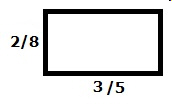a. 4/5 x 3/8 = 3/10 c. 3/5 x 2/8 = 3/20 b. 3/5 x 2/8 = 1/5 d. 4/5 x 1/4 = 2/10

4.

What equation does this model represent?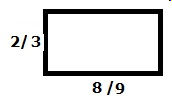a. 2/3 x 1 = 2/3 c. 2/3 x 8/9 = 16/27 b. 3/6 x 7/9 = 15/27 d. 3/3 x 8/9 = 24/27

5.

Which equation below does this model represent?a. 1/2 x 4/5 = 3/5 c. 3/6 x 7/10 = 1/3 b. 3/6 x 8/10 = 3/10 d. 3/6 x 4/5 = 2/5

6.

What equation does this model represent?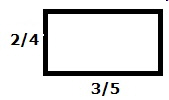a. 1/2 x 1/2 = 3/10 c. 2/4 x 6/10 = 7/20 b. 2/4 x 3/5 = 2/5 d. 2/4 x 3/5 = 3/10

7.

Which equation below does this model represent?a. 4/7 x 2/6 = 4/21 c. 4/6 x 1/3 = 1/4 b. 3/7 x 1/3 = 1/2 d. 4/7 x 3/6 = 2/7

8.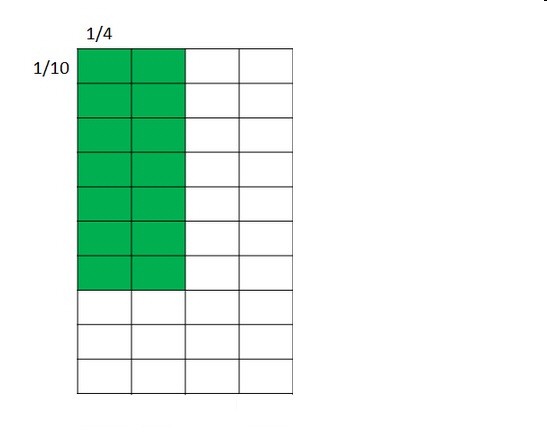Which equation does the green area of this model represent?
 a. 3/5 x 1/2 = 7/10 c. 7/10 x 2/4 = 7/20 b. 5/10 x 1/2 = 1/4 d. 6/10 x 1/2 = 7/10

9.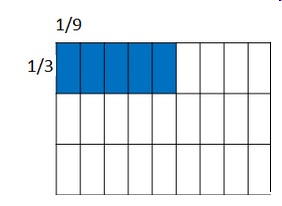Which equation below does blue section of this model represent?
 a. 5/9 x 2/3 = 4/27 c. 1/3 x 5/9 = 6/27 b. 1/3 x 5/9 = 5/27 d. 1/4 x 4/9 = 5/27

10.What equation does this model represent?
 a. 1/2 x 2/7= 4/14 c. 2/4 x 3/6 = 1/4 b. 2/5 x 3/7= 6/35 d. 2/4 x 3/7 = 3/14